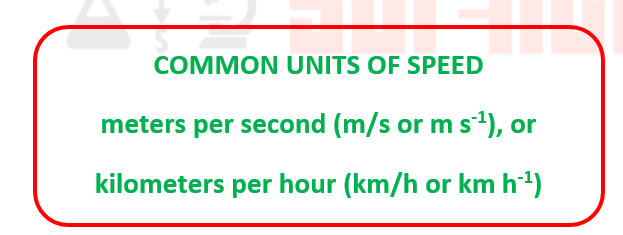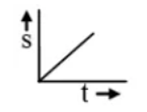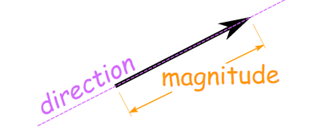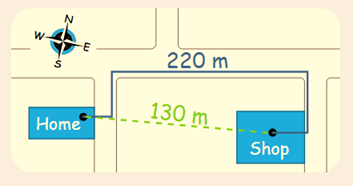### SPEED AND VELOCITY

Fun with science > Knowledge Hub > Science > Physics > SPEED AND VELOCITY

# SPEED AND VELOCITYSPEED

Speed is a scalar quantity which refers to “how fast an object is moving.”

Speed= Distance/Time = s/t

·     s means distance (“s” for “space”)

·     t means time

Example:
You run 360 m in 60 seconds.

Speed =s/t

=360 m/60 seconds

=6 m/1 second

So your speed is 6 meters per second (6 m/s).CONSTANT SPEED

When the speed does not change it is constant.

For constant speed, the speed at any instant will remain same.VELOCITY

Velocity is speed with a direction.

It is actually a vector as it has magnitude(Value) and direction.Velocity = Displacement/Time in a direction.

Example:

You walk from home to the shop in 100 seconds, and the shop is around 220 metres from the house. what is your speed and what is your velocity?Speed
= 220 m/ 100 s = 2.2 m/s

Velocity
= 130 m / 100 s East = 1.3 m/s East

BOOKS

We have our e-books published on Amazon for Grade 3 and Grade 4. The books serve as an important guide for Science Olympiads organized by SOF, Silverzone, Unified Council and others. Books are designed to help students understand key science concepts.

The key highlights of the book are:

·      Well explained topics

·      Use of diagrams and images for students to visualize

·      Test exercise after each chapter for self-assessment and evaluation

·      Interesting facts sections spread across the book